Interactive Visualization of a Approximations to Different Points on a Surface.

Example function of two variables: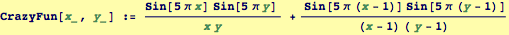Plot this function over suitable range of the variables x and y: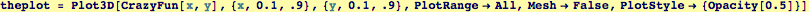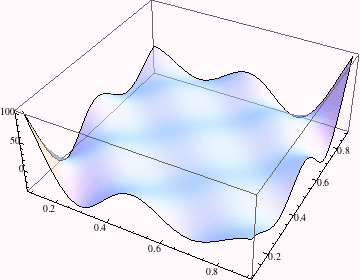Now, approximate the function about a specific point (xo, yo), using Mathematica's Series function (this is now a function of four variables)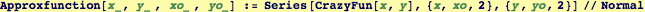and plot the approximate function in the neighborhood of (xo, yo):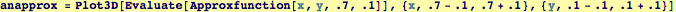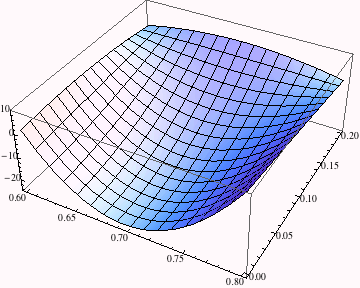Both the original and the approximate function can be plotted simultaneously: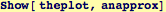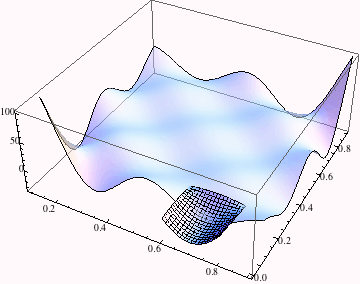This final little bit selects random points (xo, yo) and fits Taylor expansions at different points, then displays them individually as well as with a superposed plot of the original function. Here we fix the evaluation points randomly.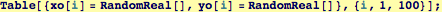The next function automates the small approximating surface patch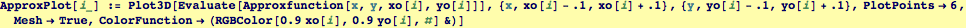To build a sequence of graphics, I'll build a stack of ten graphics objects by using a recursive method. The next command sets the end of the recursion loop.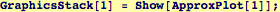Here is the recursive function.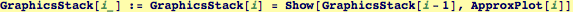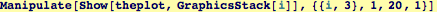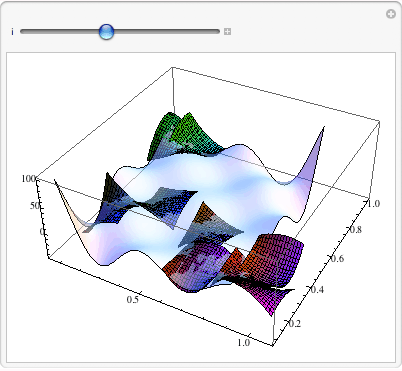Created by Wolfram Mathematica 6.0  (20 September 2007)